﻿ 多功能腰颈椎电动康复牵引床智能控制系统研究

# 多功能腰颈椎电动康复牵引床智能控制系统研究Research of Multi-Functional Lumbar and Cervical Spine Electric Rehabilitation Traction Bed Intelligent Control System

Abstract: In view of a single traction way of the traditional lumbar and cervical spine traction equipment, the treatment of patients with different symptoms cannot be satisfied, and the intelligent control system of multi-functional lumbar and cervical spine electric rehabilitation traction bed was put forward. The system adopts the closed-loop control mode, receives the input of touch screen and tension sensor under the control of the microcontroller, and achieves traction activities by the traction drive mechanism with motor. The input parameters and operating status are displayed at the same time, and it is a traction device with digital touch screen user interface. The user can set the static (continuous), intermittent and cyclic traction functions which have hold time, rest time and treatment time based on needs, and can display the traction working status with the coordinate map in real time. By setting the duration time and the size of tension, lumbar and cervical spines are in the traction state by continuous traction bed, in order to achieve the treatment of lumbar and cervical spine disease. The experimental results have shown that the traction device has not only good dynamic response performance, precise control of traction, good human-computer interaction, patient information storage and dynamic traction, but also has good therapeutic effect, and the traction process is more stable, safe and reliable.

1. 引言

2. 系统总体方案设计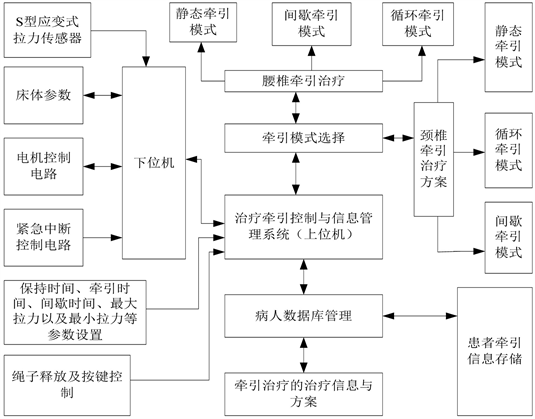Figure 1. Overall structure of electric rehabilitation traction bed control system

3. 系统硬件电路设计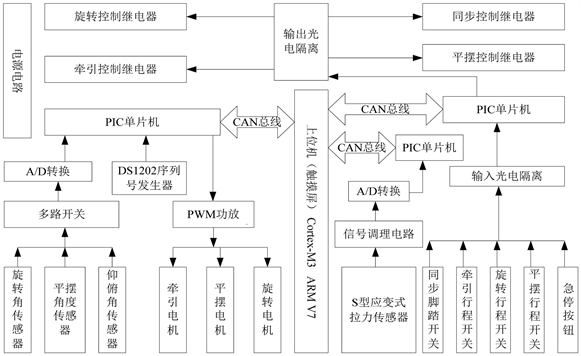Figure 2. System hardware circuit structure

4. 牵引电机控制策略设计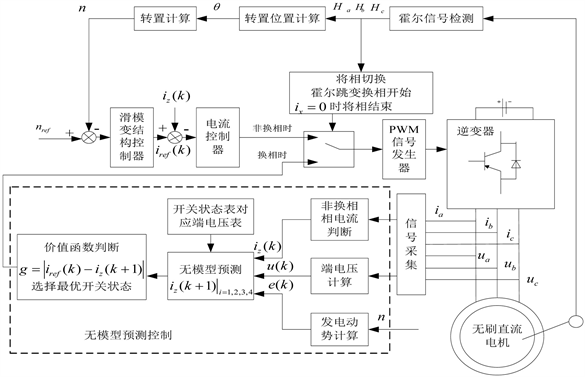Figure 3. BLDCM control strategy flow

4.1. 滑模变结构控制策略设计

$y=b{x}_{1}+{x}_{2}$ (1)

$\left\{\begin{array}{c}{x}_{1}={n}_{ref}-n\\ {x}_{2}={\stackrel{˙}{x}}_{1}=-\stackrel{˙}{n}\end{array}$ (2)

$\left\{\begin{array}{c}{\stackrel{˙}{x}}_{1}=-\stackrel{˙}{n}\\ {\stackrel{˙}{x}}_{2}=-\frac{{K}_{T}}{J}\stackrel{˙}{i}\end{array}$ (3)

$n={n}_{ref}-c{e}^{-bt}$ (4)

$f={f}_{eq}+{f}_{sw}$ (5)

$\stackrel{˙}{y}=b{\stackrel{˙}{x}}_{1}+{\stackrel{˙}{x}}_{2}=b{x}_{2}-\frac{{K}_{T}}{J}\stackrel{˙}{i}$ (6)

$\stackrel{˙}{y}=0$ 时，可求得等效控制部分函数控制率为：

${f}_{eq}=J/{K}_{T}\int \left(\text{d}{x}_{2}\right)\text{d}t$ (7)

$f=\frac{J}{{K}_{T}}\int \left[ks+\epsilon sign\left(s\right)+{x}_{2}\right]\text{d}t$ (8)

4.2. 无模型预测控制算法设计

${u}_{x0}+{u}_{y0}-2{u}_{z0}=-3R{i}_{z}-3{L}_{s}\frac{\text{d}{i}_{z}}{\text{d}t}+\left({e}_{x}+{e}_{y}-2{e}_{z}\right)$ (9)

$\left(1+R{T}_{s}/{L}_{s}\right)\left[{i}_{z}\left(k+1\right)-{i}_{z}\left(k\right)\right]-\left[{i}_{z}\left(k\right)-{i}_{z}\left(k-1\right)\right]=\frac{{T}_{s}}{3{L}_{s}}\left\{\left[e\left(k+1\right)-e\left(k\right)\right]-\left[u\left(k+1\right)\right)-u\left(k\right]\right\}$ (10)

$\left[{i}_{z}\left(k+1\right)-{i}_{z}\left(k\right)\right]-\left[{i}_{z}\left(k\right)-{i}_{z}\left(k-1\right)\right]=\phi \left(k\right)×\left\{\left[{i}_{z}\left(k\right)-{i}_{z}\left(k-1\right)\right]-\left[{i}_{z}\left(k-1\right)-{i}_{z}\left(k-2\right)\right]\right\}$ (11)

$\phi \left(k\right)=\left[e\left(k+1\right)-e\left(k\right)\right]-\left[u\left(k+1\right)-u\left(k\right)\right]/\left[e\left(k\right)-e\left(k-1\right)\right]-\left[u\left(k\right)-u\left(k-1\right)\right]$ (12)

${i}_{z}\left(k+1\right)=\phi \left(k\right)\left[{i}_{z}\left(k\right)-2{i}_{z}\left(k-1\right)+{i}_{z}\left(k-2\right)\right]+\left[2{i}_{z}\left(k\right)-{i}_{z}\left(k-1\right)\right]$ (13)

5. 压力传感器的数据融合算法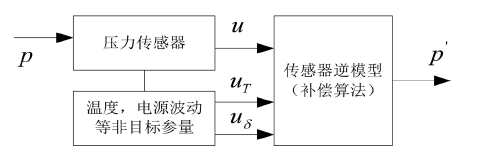Figure 4. Sensor data fusion system principle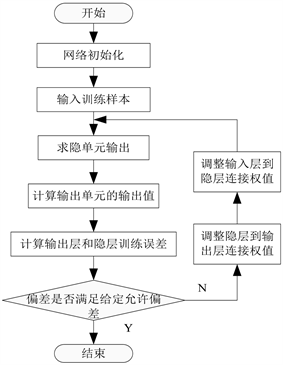Figure 5. BP neural network training process and its algorithm

6. 实验结果与分析

6.1. 无刷直流电机控制策略性能分析Table 1. Brushless DC motor related test parameters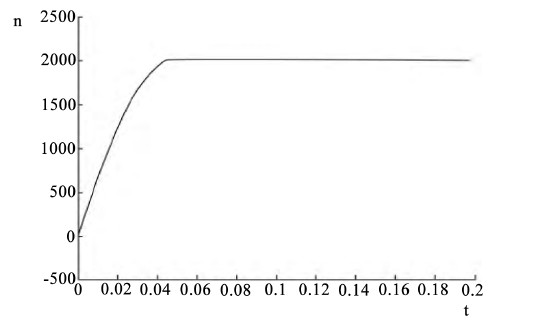Figure 6. The speed change waveform under the control of sliding mode variable structure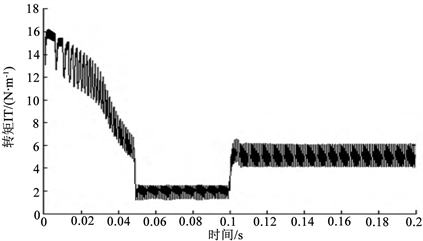Figure 7. Variation of electromagnetic torque under the control of sliding mode variable structure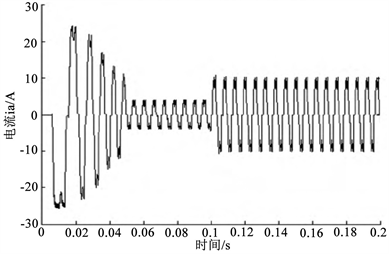Figure 8. The phase current variation waveform under the control of sliding mode variable structure

6.2. 数据融合结果及性能分析

6.3. 系统测试与分析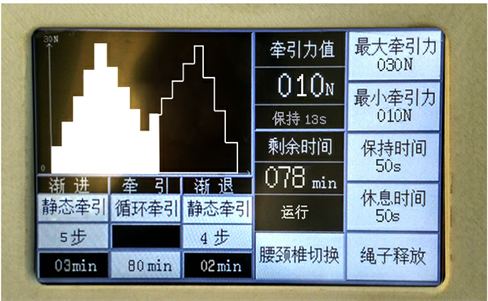Figure 9. System run effect chart

7. 结束语

 郭子豪. 基于LabVIEW和CAN总线的多功能颈椎牵引床控制系统设计[D]: [硕士学位论文]. 长春: 吉林大学, 2016.

 茹意. 多功能智能化牵引床系统设计[D]: [硕士学位论文]. 成都: 电子科技大学, 2010.

 王田田. 多功能智能颈椎康复治疗仪的研究[D]: [硕士学位论文]. 武汉: 华中科技大学, 2013.

 Raja, R.A., Khemani, V.D., Lakhair, M.A., et al. (2012) Discectomy in Single Level Lumbar Disc Disease. Journal of Ayub Medical College Abbottabad, 24, 1131-1135.

 Autio, R.A., Karppinen J., Niinimki, J., et al. (2006) Determinants of Spontaneous Resorption of Rote Rvertebral Disc Hermiations. Spine, 31, 1247-1252.
https://doi.org/10.1097/01.brs.0000217681.83524.4a

 孔群. 随动慢牵治疗仪的控制与信息管理系统[D]: [硕士学位论文]. 济南: 山东建筑大学, 2015.

 刘健. 多功能腰椎间盘康复治疗装置的研制[D]: [硕士学位论文]. 哈尔滨: 哈尔滨工业大学, 2015.

 仲为武. 三维牵引床控制系统设计[D]: [硕士学位论文]. 济南: 山东大学, 2006.

 姜卫东, 黄辉, 王培侠, 张忠. 基于图解法的无刷直流电机抑制换相转矩脉动的方法[J]. 中国电机工程学报, 2016, 36(15): 4258-4265.

 史婷娜, 李聪, 姜国凯, 夏长亮. 基于无模型预测控制的无刷直流电机换相转矩波动抑制策略[J]. 电工技术学报, 2016, 31(5): 54-61.

 崔臣君, 刘刚, 郑世强. 基于线反电动势的高速磁悬浮无刷直流电机无位置换相策略[J]. 电工技术学报, 2014, 29(9): 119-128.

 柴俊, 李坤, 过磊. 无刷直流电机滑模变结构控制策略研究[J]. 齐鲁工业大学学报, 2016, 30(4): 55-59.

 郭鸿浩, 周波, 左广杰. 无刷直流电机反电势自适应滑模观测[J]. 中国电机工程学报, 2011, 31(21): 142-149.

 康忠健, 陈学允. 非线性扩张状态观测器的一种设计方法[J]. 电机与控制学报, 2001, 5(3): 199-203.

 刘二林. 基于BP神经网络的压力传感器数据融合研究[J]. 工业仪表与自动化装置, 2012(4): 7-11.

 韦以明. 基于神经网络的传感器数据融合处理[J]. 数据采集与处理, 2006(21): 218-221.

Top